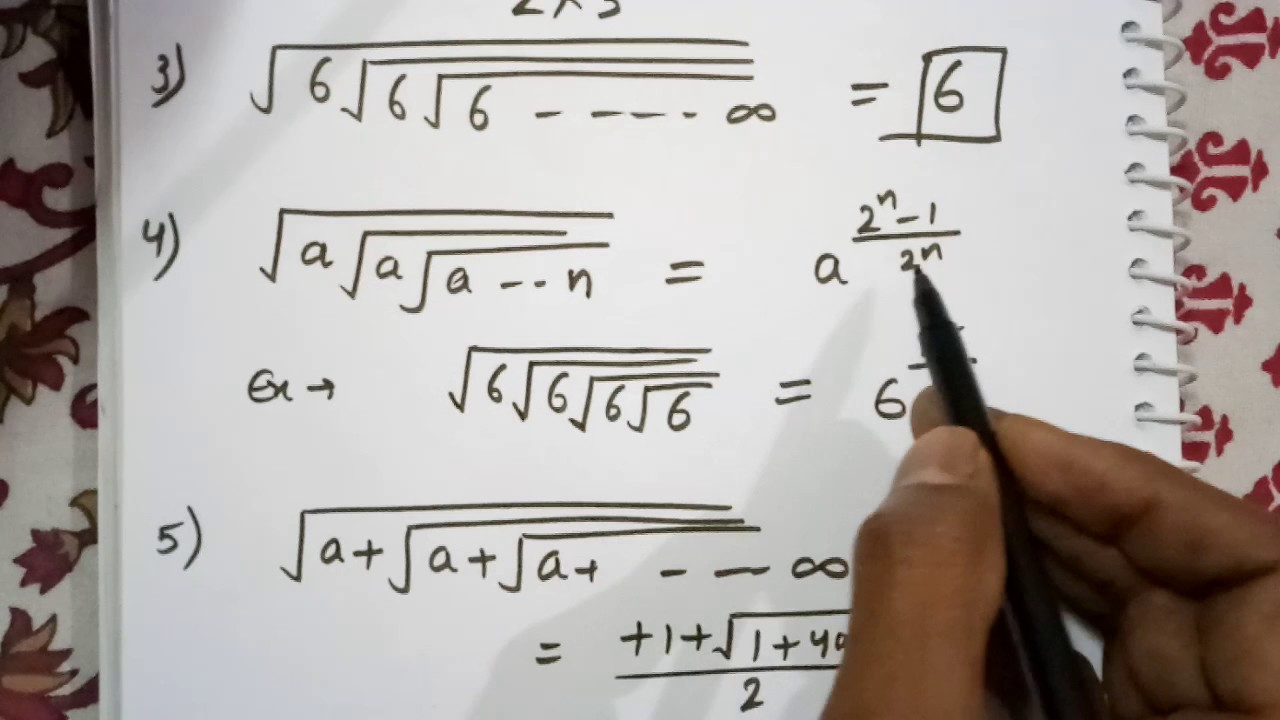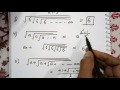Maths coaching for govt jobs competitive exams - Welcome to Coaching123.in > One stop place for govt jobs preparation and govt jobs news. In the previous class SET 1 we have discussed Maths 22 important questions asked in previous govt jobs like SSC (staff selection commission) and Indian railways.

* If you have missed the SET 1, then you can read it here - Click here

In this part SET 2, we will cover few more important Maths questions and answers based on previous year govt jobs pattern. You can make important notes OR you can bookmark our website www.Coaching123.in !!

So, lets start !

## Maths coaching for govt jobs competitive exams - SET 2

1. Price of an article increased twice consecutively by 10% and as a result of the article becomes Rs.484. Then the initial price of the article was ?

a. Rs.390
b. Rs.375
c. Rs.380
d. Rs.400

Ans. d

2. If Raju runs at 31.2 km/h, then in 3 sec he will run ?

a. 26 m
b. 42 m
c. 35 m
d. 12 m

Ans. a

3. A man buy car at 15/16th of its value and sells it for 10% more than its value. His gain percentage is ?

a. 15.57
b. 11.67
c. 16.67
d. 17.33

Ans. d

4. A person sold 320 oranges for the cost price of 400 oranges. His profit percentage is ?

a. 20%
b. 25%
c. 11.5%
d. 15%

Ans. b

Explanation -

SPX320 = CPX400

SP/CP = 400/320

SP/CP = 5/4

Therefore,

SP = 5
CP = 4

Profit = 5-4=1
Profit percentage = 1/CPX100 = 1/4X100 = 25%

5. A four digit number is divisible by 7 becomes divisible by 3 when 10 is added to it. The largest such number is ?

a. 9987
b. 9989
c. 9996
d. 9999

Ans. d

6. The HCF of two numbers is 9 and their LCM is 270. If the sum of the number is 99, their difference is equal to ?

a. 15
b. 12
c. 18
d. 9

Ans. d

7. The monthly salaries of A, B and C are in ratio 2:3:5. If the salary of B and C together is Rs.8000. Then the percentage of salary of C more than that of A ?

a. 200%
b. 150%
c. 100%
d. 75%

Ans. b

8. Average of 3 numbers is 21 and the average of these 3 numbers and a fourth number is 18. The 4th number is ?

a. 8
b. 9
c. 8.6
d. 1

Ans. 9

Explanation -

18x4-21x3 = 72-63 = 9

9. The average of 11 numbers is 6. If each number is multiplied by 7, then the average of the new set of number is ?

a. 36
b. 66
c. 77
d. 42

Ans. d

10. A train 150m long crosses a standing man in 5 sec. The speed of the train is ?

a. 108 kmph
b. 40.5 kmph
c. 112 kmph
d. 32 kmph

Ans. a

11. The number 77 is divided into 2 parts such that the HCF of two parts is 11 and their LCM is 110. The smaller of the two parts is ?

a. 44
b. 55
c. 11
d. 22

Ans. d

12. 2/3rd of a number is greater than 1/6th of it by 10. The number is ?

a. 15
b. 20
c. 12
d. 33

Ans. b

-----------------------
सरकारी नौकरी में आने वाले सामान्य ज्ञान प्रश्नोत्तर की तैयारी के लिए हमारे वेबसाइट www.Coaching123.in के साथ बने रहे!
Maths coaching - Govt jobs Previous asked questions - SET 2Reviewed by ani on 1:25 AM Rating: 5